Electrics Index

Direct Current

Introduction..... Nomenclature..... DC Generators..... DC Electric Motors.... Cells , batteries , accumulators.... Rectifiers.... Resistance....

Introduction

This page includes notes on direct current devices including generators, batteries, motors, rectifers, resistances etc.

A direct current circuit is one in which the current flow is in one direction as opposed to an alternating current which is generally sinusoidal.   Direct current is used to power electronic devices, all portable electric audio, video and photgraphic equipment, most electrolytic chemical processes, electric arc furnaces, electric motors, and rail networks, etc etc. DC power is obtained from a number of sources a - list of some sources is provided below in order of popularity

 Batteries/Cells - Chemical Action Rectified AC Generators-dynamos - Mechanical energy via electro-magnetic energy transfer Solar power - photo-voltaic Fuel Cell - Chemical Action Temperature -(Seeback/Thompson effect) - used for temperature measurement

Nomenclature

B = flux density (Teslas = webers/m2)
c = Number of parallel conductor paths on armature
E = e.m.f. (volt)
e = instantaneous e.m.f. (volt)
I = current flow (amperes)
i = instantaneous current flow (amperes)
V = potential difference (volt)
v = potential difference (volt)
Nr = speed (revs/s)
l = length (m)
F = force (N)
H = magnetic field strength (Aturns/m)
L = Inductance (Henry)
T = shaft torque (newton metres)
t = time (seconds)
P = shaft power (watts)
p = Number of pole pairs
u = velocity (m/s)
Z = number of armature conductors
Φ = flux (webers)
Ψ = flux linkages (weber -turns)
μo = permeability of free space (H /m)
μr = relative permeability
μo = absolute permeability =μo.μr

DC Generators

Consider a single rectangular coil of wire rotating in an magnetic field as shown below.  The rotating coil is called the armature.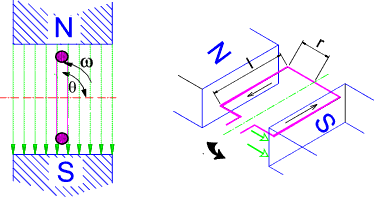The coil is rotating at a angular velocity of ω.    The coil comprises two conductors each one has a length l.    The coil has a radius r.

The direction of current flow is determined using Flemings Right Hand Rule ref Electromagnetism -Flemings RHR and it is clear that the e.m.f generated in the conductors are additive.  The total e.m.f generated in the coil is the sum of the e.m.f. generated in each conductor..

The maximum e.m.f. induced in each conductor as it moves normal to the lines of magnetic force with a velocity u(m/s) is

Em = Blu = Blωr

As the coil rotates the total instantaneous e.m.f. generated in the coil (2 conductors) is given by the equation

e = 2Blu.sin θ = 2Blωr sin θ = BAω sin θ

Note The area of the coil A = 2lr

In practice there are generally a number of conductors connected in series in the slots on the armature.
If there are n conductors in each slot then the e.m.f generated in terms of conductors (not Turns) is

Emax = 2Blnu =2Bln.ωr ..... and..... e = 2Blnu.sin θ = 2Bln.ωr.sin θ

If N is the number of turns (N =n/2) then

e = 2BNlωr sin θ = BAω sin θ

The figure below illustrates the armature voltage resulting from the progressive (ACW) rotation of the armature coil in a fixed magnetic field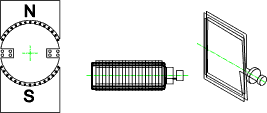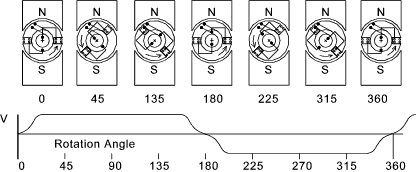Commutation

As can be seen the e.m.f induced changed direction on each half turn.  To generate an e.m.f which does not change direction the brush contacts have to be reversed each time the coil rotates through θ = π. This is done using a commutator which is simply a single ring divided in two through the diameter.  Both halves of the commutator are isolated from each other.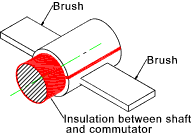As a result of commuation the output voltage is as shown below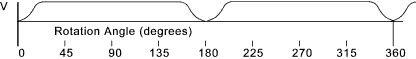Armature

In real generators the armature coils are wound onto the armature using a variety of methods.  Drum winding involves a number of coils separately wound round the armature and connected in series.   In the figure below an armature is wound such that at every instant as it rotates two equal e.m.f's in parallel exist across the brushes.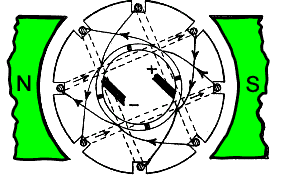Armature Drum winding

The figure below shows a 4-pole machine with and eleven slop armature core with eleven coils A-H. These coils may each have a number of turns.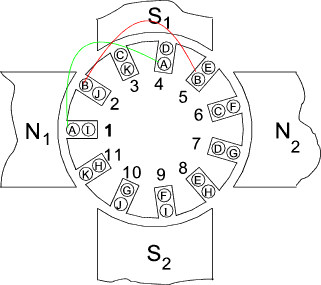A number of methods are available for connecting the coils to the commuter segments.  These can be broadly divided into two generic types as shown in the figure below.  Lap windings and wave windings.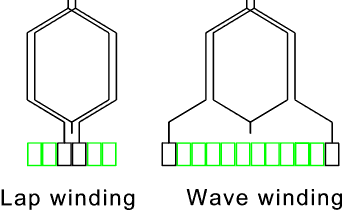In lap winding the two ends of any coil are taken to adjacent segments of the commuter and in wave windings the ends are taken to spaced segments.  A lap winding system has the same number of parallel paths as there are poles.   The wave winding system has only 2 paths in parallel.

e.m.f. generated in armature winding.

Considering a generator with N poles (N = 2.p (pole pairs) ,   if Φ is the total flux from one pole and Nr is the armature speed (revs/s) then in each revolution of the armature each conductor cuts the total flux twice.

flux cut per sec by each conductor = 2 Nr p Φ = e.m.f. in each conductor.
average e.m.f. generated in each conductor = 2 Nr p Φ

If Z = the number of armature conductors and c is the number of parallel paths (2 for wave windings and 2 p for lap windings).
Then Z/c = the number of conductors in series in each path.  The e.m.f generated is assumed to be the average e.m.f x the number of conductors in series.

E = 2 Nr p Φ. Z/c

Note: the waveform of the generated e.m.f in each conductor follows that shown in the figure above under the commutation heading.  E is the sum of a number of the individual average e.m.f. 's

Field Windings

The generator fields for very small dynamos is generally based on permanent magnets.  For the majority of industrial and utility generators the fields are produced using electomagnets.

The figure below shows a simple representation of a field winding which provides a powerful field confined to the space between the armature and the pole faces..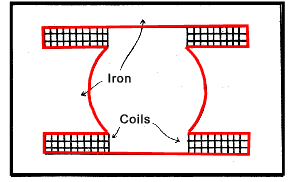Field winding

The electromagnets are often self energised (self-excited)and there are two methods of wiring the fields to this purpose: series wound fields and shunt (parallel) wound fields.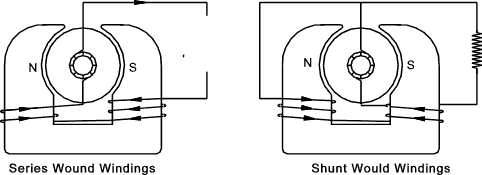For series wound fields the field coils consists of a few turns of heavy gauge wire which carry the full supply current.   When current flows in the external circuit the e.m.f across the field is practically zero.  A small current in the external circuit results in a small magnetic field and hence a small e.m.f .  Larger currents result in larger e.m.f.s but a maximum is attained when the core is magnetically saturated.  With higher currents the e.m.f of the generator drops as a consequence of the increasing p.d. across the internal resistance of the windings.  If the generator was short circuited the generator e.m.f. would fall to zero.

For shunt wound field windings the windings consist of many turns of wire the ends of which are connected to the brushes in parallel with the external circuit.   As the external current increases the p.d. across the internal resistance of the generator increases thus decreasing the terminal p.d.  If E is the generated e.m.f and Ia and Ra are the armature current and resistance and V is the terminal p.d.

V = E - IaRa

In normal operation the armature resistance Ra is small and the terminal voltage remains reasonably constant.  There is a tendency of the terminal voltage to fall off with increasing current.  This can be offset by having a shunt wound generator with a few turns of series winding of thick wire.   This arrangement, called a compound wound machine, produces a virtually constant supply voltage....

DC Electric Motors

When a D.C generator armature is rotating the current flowing results in an induced magnetic flux which tends to oppose the motion.   If this current is from an external source the induced flux would result in a torque tending to cause motion.

A rotating electric motor also still acts as a generator and generates an e.m.f. opposing its motion.   This is called the back e.m.f . The back e.m.f (E) for each each conductor as it passes through the magnetic field is given by.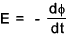Each conductor on the armature of a motor contributes to the torque a force which is given by the formula

F = B.I.l

B = Flux in Webers, I =current in amperes, l = length of the conductor (m).  The direction of the force is found by applying Flemings Left Hand Rule. (Flemings Left Hand Rule ) The principle of the action of the electric motor is shown below.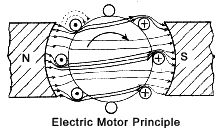When the current flows in the armature conductors of a motor (or generator) a torque on the motor shaft results. (in a generator to torque is a reactive torque which must be overcome by the driver).  This torque is T (Newton --metres).   If the rotation speed of the shaft is N (revs/s) then the power (P -watts)developed by the motor =

P = 2.π.N.T

If the e.m.f across the armature conductors is E (generator e.m.f or motor back e.m.f) and the current in the armature windings = I a the electrical power in the armature =

P = E.I a = 2.π.N.T
Therefore T = E.I a / (2.π.N )

However E is proportional to the rate of change of flux cut by the armature conductors.  This depends on the flux density over the pole faces.

The poles for a motor are generally electromagnets which can be series wound or shunt wound (similar to a generator).  The two arrangements are shown schematically in the figure below.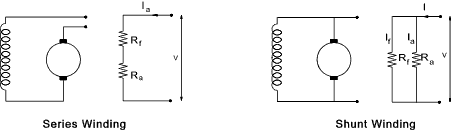A series wound motor in arranged in a similar way to a series wound generator.  The applied voltage V drives a current of Ia through the field and armature windings while a back e.m.f. of E is generated by the rotating armature. The formula for the applied voltage is

V = Ia(Ra + Rf) + E

On start up the series motor, the value of E is small and therefore Ia is large.   Since the flux density of the field is roughly proportional to the field current.   At this stage the following relationship applies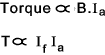However as the field current is the same as the armature currentTherefore when a high starting torque is needed a series wound motor is suitable because Ia is large and therefore T is very large.

A series wound motor should always be loaded .   If, when operating, the load in removed ( due to say a coupling or chain failure) , the power absorbed will decrease .  The field current will then reduce resulting in a reduction of the magnetic field.  The back e.m.f. is given by

E = V - Ia ( R a + R f )

and V is constant.   Therefore E will increase which follows from the motor speeding up.  This increase compensates for the decrease in flux so that the smaller the armature current the greater the motors speed until the armature, if unable to provide enough centripetal forces, breaks up.

To reverse the series wound motor, either the current in the field windings or the current in the armature windings must be reversed.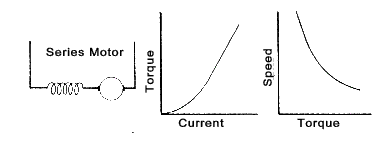The shunt wound electric motor has the following relationship

V = E + Ia.R a

In a shunt wound motor the field is constant as it connected directly across the supply.   The speed therefore does not rapidly increase on removing the load.  On the other hand the starting torque is smaller than on the equivalent series wound machine since .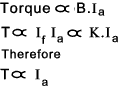It has been shown above that T = E.I a / (2.π.N ) and therefore T.N = K. E.I a.........[K = 1/(2.π)]
as E is proportional to N in a constant flux then , at a speed N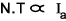From this it is clear that a shunt wound motor tends to be a constant speed machine...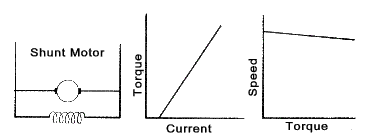A series wound machine with an additional shunt winding, called a compound motor, has benefits of the series wound motor (high starting torque) with the benefit of the shunt motor (constant speed).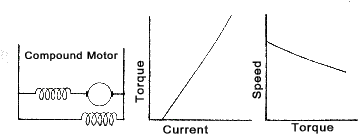Cells, Batteries, Accumulators

A cell is a device in which chemical energy is directly converted to electrical energy.  It comprises of two half cells connected in series by the conductive electrolyte.   A battery consists of one or more cells in series.    Each cell has a positive terminal, shown on diagrams by a long line, and a negative terminal, shown by the shorter thicker line.   These do not touch each other but are immersed in a solid or liquid electrolyte.

The electrolyte is a conductor which connects the half-cells together.   It also contains ions which react with chemicals of the electrodes.   Chemical energy is converted into electrical energy by chemical reactions that transfer charge between the electrode and the electrolyte at their interface.   These reactions result in a current flow through the cell.   Ordinary, non-charge-transferring reactions also occur at the electrode-electrolyte interfaces.   These non-faradaic reactions are one reason that cells (particularly the lead-acid cell of ordinary car batteries) "run down" when sitting unused.

Cells which are only used once until the chemical action is depleted are called primary cells and are generally dry type.  Cells which can be re-used, after recharging by driving a current through them in the reverse direction, are called secondary cells.   Vehicle batteries are generally constructed using wet secondary cells (accumulators).

Cell and Battery Ratings
The capacity of a cell/battery is the amount of charge available in ampere-hours (Ah).   The capacity of a cell/battery is related to the quantity of electrolyte and the surface area of the plates.   A automobile rated for 200Ah will deliver 20 amperes for 10 hours.  The battery capacity is often also given a C rating e.g. a C/10 rating for a battery is the rate of amperes which can be delivered in 10 hours.  A C/10 rating of 20 is effectively a 200 Ah rating based on a 10 hour discharge time.  Seondary batteries are generally available with C/8, C/10 and C20 ratings.

Primary Cells
The simplest primary cell consists of a zinc plate and a copper plate immersed in a dilute sulphuric acid electrolyte.

The zinc plate on immersion loses some of its surface molecules as positive ions(++Zn) to form ZnSO4 leaving two electrons behind causing the plate to become negatively charged.   There is a tendency for the plate to attract the ions back but a equilibrium is attained with a net negative charge on the plate.

The copper plate when immersed loses negative copper ions with an equilibrium being reached with the plate at a negative potential relative to the electrolyte.

The result of a zinc and a copper plate being inmmersed in the same electrolyte is that a the copper electrode has net positive potential of about 1,1 V relative to the zinc electrode.  This p.d. is a measure of the electro motive force of the cell.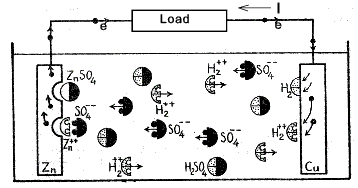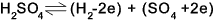As current flows (electrons drift) through the external load the zinc becomes less negative resulting in it attracting more sulphate ions.
The zinc and sulphate ions neutralise each other and go into solution as zinc sulphate thus allowing more zinc molecules to ionize and more electrons to flow from the zinc plate through the external load.

Over time the acid becomes weaker and hydrogen bubbles of the copper plate.  In external load the conventional current flows from the copper to the zinc and in the solution it effectively flows from the zinc to the copper.

Over time the chemical /electrical activity reduces as the zinc goes into solution and a layer of hydrogen gas coats the copper plate.

The above notes relate to the principle of operation of primary cells.   There are currently many types of primary (non-rechargeable) cells and batteries available some are listed below.

Primary Cell types

 Alkaline Currrently the most widely used battery type Carbon Zinc Has been in use over long time period MercuricOxide< Hazardous as waste item SiverOxide Lithium, Small 9V or less often button type labeled Li or Cr Lithium, Large More than 9 V : Zinc Air Usually Button sized

Secondary Cells
A secondary cell is one which can be recharged many times by passing an electric current through it in the reverse direction to that for normal operation and reversing the chemical process.  The most largely used high power secondary cell is the lead acid cell.  A single lead-acid cell can develop an e.m.f under load of about 2V. A discharged lead-acid cell has a potential difference of about 1,75V dependng on the rate of discharge.  The electro-chemical process involved in the lead acid cell is simply described below.

If two lead plates are immersed in dilute sulphuric acid the surface of each becomes coated with a trace of lead sulphate.  When current is passed through this cell, the lead sulphate on the anode is changed to lead peroxide and that on the cathode to metallic lead.   In this condition the cell has an e.m.f. of about 2,1 volts.   If the cell is discharged , both plates again become coated with lead sulphate.   Continual charging and recharging increases the thickness of these chemical active layers, and as a result the capacity of the cell.  This process of plate forming is expensive and is not used commerically.

Normally the lead acid plates consist of lead-antimony alloy grids packed with a paste made from litharge (PbO), red lead (Pb3O4) and sulphuric acid.   When the cell is first charged the whole thickness of the past changes: that on the anode becomes lead peroxide and that on the cathode becomes spongy lead.  The grid is unaffected.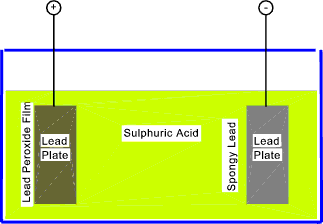This type of cell has a large capacity and is mechanically robust.
The chemical reactions taking place during charging are

Negative Plate (Cathode)....PbSO4 + H2++ --> H2SO4 + Pb

Positive Plate(Anode)....PbSO4 + SO4- - + 2H2O --> 2H2SO4 + PbO2

During this process the sulphuric acid becomes more concentrated (increased specific gravity from 1,12 to 1,26 is small accumulators and 1,17 to 1,21 in large units).  The positive grid becomes filled with lead peroxide which is chocololate brown in colour and the negative grid becomes filled with spongy lead (silver).

On discharge the following reactions occur.

Negative Plate (Cathode)....Pb + SO4- - --> PbSO4

Positive Plate(Anode)....PbO2 + H2++ + H2SO4 --> PbSO4 + 2H2O

They plates become grey in colour, the e.m.f reduces and the specific gravity of the sulphuric acid falls.

The above notes relate wet lead acid type.   There are in practice a number of different types as listed in the table below

Secondary Cell Types
 Gel battery: Developement of lead acid with gell replacing liquid electrolyte -(VRLA) Absorbed glass mat: Development of lead acid type with electrolyte soaked into glass fibre mat.   Flat or spiral wound plates..-(VRLA) Rechargeable Alkaline< Now rarely used. Nickel-iron battery Nickel Cadmium (NiCd) cells These have low capacity (but with large number of recharge cycles) Nickel-Metal Hydride (NMh) Are being developed to have high power density is small cell sizes Lithium-ion (Li-ion) High power capacity in relatively small cell sizes Super_iron battery Under developement Molten salt battery: A modern development with high power densities uses high temperature molten salt technology. This type of battery is considered a future hope for electric vehicles.

Note :VRLA stands for valve regulated lead acid and is the designation for maintenance-free lead-acid batteries.   VRLA batteries types include Absorbent glass mat battery , and Gel batteries.  These batteries are often designated as sealed lead-acid batteries, but this term not exactly correct.   A sealed battery would be a safety hazard due to overpressure risks when overcharging, and there is always a safety valve present, hence the name valve-regulated.

Rectifiers

A large part of the DC systems used in industrial and domestic systems are rectified AC system.  AC supplies are available everywhere and therefore it is most convenient to use these existing supplies to power DC systems such as battery chargers, portable radios etc.

Most rectifiers are based on the diode which is a device that passes current in one direction only.  Use of these items allow the conversion of alternating current supplies to direct current supplies.  The diodes can be used to produce half wave rectified current and full wave rectified current. These rectified currents are unidirectional but they still have a sinusoidal waveform.  To produce a steady current flow as is required for most applications smoothing circuits are required.

Half Wave Rectifier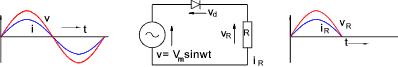Assuming the diodes have zero forward resistance and infinite back resistance then the instantaneous, values of the resulting rectified waveform are.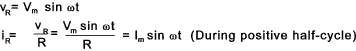The rectified voltage and current mean and RMS values are shown below.
The methods of deriving these values are shown on webpage Av_RMS Calculation.
to determine the mean value the plot of the rectifed variable is integrated from 0 to π to determine the area then divided by 2π.
to determine the RMS value the plot2 is integrated from 0 to π to determine the area then divided by 2π.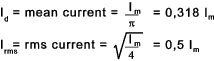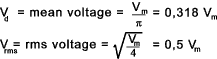Full Wave Rectifier

The full wave rectifier circuit shown is called a bridge rectifier network...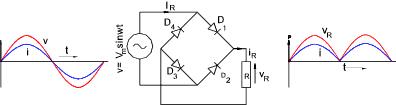Assuming the diodes have zero forward resistance and infinite back resistance then the instantaneous, values of the resulting rectified waveform are.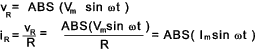The rectified voltage and current mean and RMS values are shown below.
The methods of deriving these values are shown on webpage Av_RMS Calculation.
to determine the mean value the plot of the rectifed variable is integrated from 0 to π to determine the area then divided by π.
to determine the RMS value the plot2 is integrated from 0 to 2.π to determine the areas2 then divided by 2π.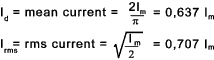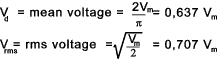The rectifier circuits produce a voltage and current flow which is unidirectional but the variables still have a large variation in the form of a half sinusoidal pattern.   Many systems require a continuous steady voltage/current to operate successfully.  To achieve this a smoothing system is required.  A very simple anology to an electical smoothing circuit is a hydraulic accumulator or reservoir system.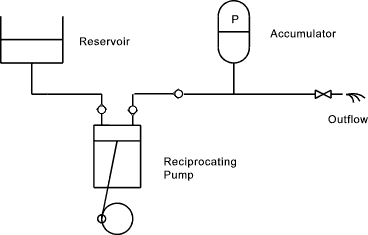The above simple hydraulic system illustrates that that the reciprocating pump produces a fluctuating flow of approximately sinusoidal flow rate with no flow on its charging stroke.   The accumulator pressurises when the pump is at full flow and discharges to the system when the pump outlet falls.    This significantly smooths the flow such that the outflow is relatively continuous.  A valve which adds resistance to the outflow helps with this smoothing process.

The method of producing smoothing in a electrical circuit is to use capacitors as shown below.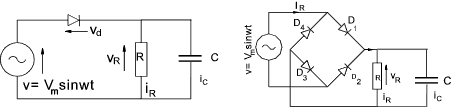Enhanced smoothing results from restricting the varying voltage component.  This is achieved by including a cored inductance in series with the capacitor/load system as shown below.  Having two capacitors with an inductance provides yet another stage of smoothing.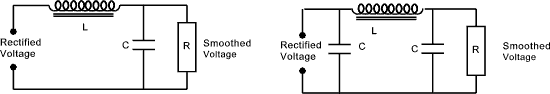The above notes relate to the popular semi-conductor rectifier diode types but other types of rectifier are also using in industry including.

a) Using AC motors to drive DC generators
b) Using AC motors to continuously reverse contactors
c) Mercury Arc Rectifiers

More detailed notes on different types of rectifiers are found in the linked websites

Resistance

The electrical resistance is a measure of the degree to which an material opposes the passage of an electric current.   The SI unit of electrical resistance is the ohm.   Its reciprocal quantity is electrical conductance measured in siemens.  If a conducter had zero reisistance then if an e.m.f. of any value where applied across its ends there would be an infinite current.   It is equivalent to friction in mechanical engineering.

The ohm is the electrical resistance of a conductor in which a constant current of 1 ampere flows under a constant voltage of 1 volt.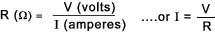A material which permits the free passage of current is called a conductor and one which opposes the passage of current called insulators.   The energy lost as a result of resistance results in the generation of heat.   This is very important in the rating of conductors and affects the selection of insulating materials.  The resistance of a material is directly proportional to the length of the conducting path and in inversely proportional to its area..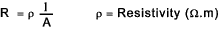A table of various resistivity values is included in Resistivity /Temperature Effects
A table identifying the resistivity of various insulators is provided below

 Insulator Ro @ 0 deg C Ohms. m Bakelite 1 Glass 5.109 to 10 13 Marble 108 Mica 1011 to 1015 Paraffin oil 1016 Paraffin wax 1016 Perspex 1013 Polystryrene 1014 to 1016 Porcelain 1012 to 1013 Pressed amber 1016 Vulcanite 1014 Distilled Water 105

Note:     1 Ω.m = 10 6Ω mm 2 /m

 Useful relevant Links Electric Motor... Notes on how electric motors work. tpub -Notes on Direct Current Generators... Useful Power Calculations Hyperphysics - DC systems... Easy to follow information School for champions =_Direct current ...Easy to follow notes - with audio Engineers Edge ...Battery Knowledge Page- Lots of useful notes Wikipedia ...Rechargeable Batteries - Very useful reference information Rectification ...Experiment Notes and very useful moving graphics Electric motors and generators... An excellent informative site on motors and generators All about circuits. ...Free on-line set of books covering the whole subject of electricity -great

Electrics Index